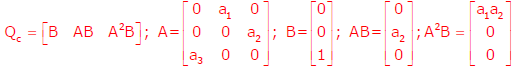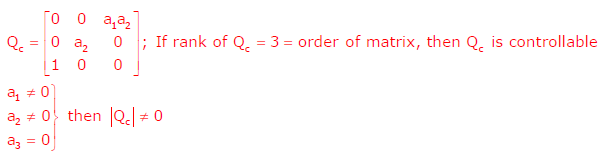# Signals and Systems - Online Test

Q1. A zero mean random signal is uniformly distributed between limits –a and +a and its mean square value is equal to its variance. Then the r.m.s value of the signal is
Explaination / Solution:Q2.
A three – bus network is shown in the figure below indicating the p.u. impedances of each element.The bus admittance matrix, Y – bus, of the network is
Explaination / Solution:Q3. The response h(t) of a linear time invariant system to an impulse δ(t) , under initially relaxed condition is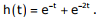The response of this system for a unit step input u(t) is
Explaination / Solution: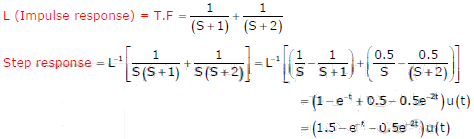Q4. The input voltage given to a converter is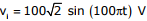The current drawn by the converter isThe input power factor of the converter is
Explaination / Solution: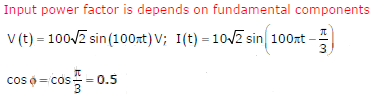Q5. The input voltage given to a converter isThe current drawn by the converter isThe active power drawn by the converter is
Explaination / Solution:Q6. Given that f(y) = | y | / y, and q is any non-zero real number, the value of | f(q) - f(-q) | is
Explaination / Solution: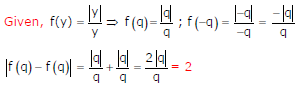Q7. An RLC circuit with relevant data is given below.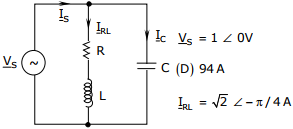The power dissipated in the resistor R is
Explaination / Solution: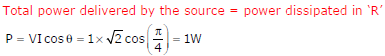Q8.
A periodic voltage waveform observed on an oscilloscope across a load is shown. A permanent magnet moving coil (PMMC) meter connected across the same load reads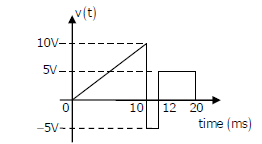Explaination / Solution:Q9. An RLC circuit with relevant data is given below.The current Ic in the figure above is
Explaination / Solution: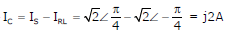Q10. The state variable description of an LTI system is given by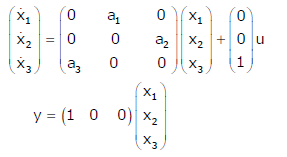where y is the output and u is the input. The system is controllable for

Explaination / Solution: